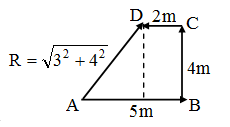# Physics

### Chapter : 1. Motion

#### Examples on Distance & Displacement

Example : 1
A person travels a distance of 5 m towards east, then 4 m towards north and then 2 m towards west.
(i) Calculate the total distance travelled.
(ii) Calculate the resultant displacement.
Solution. (i) Total distance travelled by the person = 5 m + 4 m + 2 m = 11 m
(ii) To calculate the resultant displacement, we choose a convenient scale, where 1 cm represents 1 m. We draw a 5 cm long line AB towards east and then 4 cm long line BC towards north. Finally, a 2 cm long line CD towards west. The resultant displacement is calculated by joining the initial position A to the final position D. We measure AB = 5 cm.
Since 1 cm = 1 m
∴ 5 cm = 5 m
Hence, the displacement of the person = 5m towards AD.Example : 2
A body is moving in a straight line. Its distances from origin are shown with time in Fig. A, B, C, D and E represent different parts of its motion. Find the following :
(i) Displacement of the body in first 2 seconds.
(ii) Total distance travelled in 7 seconds.
(iii) Displacement in 7 secondsSolution. (i) Displacement of the body in first 2s = 40m
(ii) From t = 0 to t = 7 s, the body has moved a distance of 80 m from origin and it has again come back to origin. Therefore, the total distance covered = 80 × 2 = 160 m
(iii) Since the body has come back to its initial position, the displacement is zero.